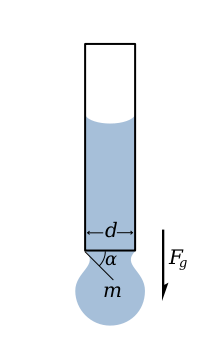# Surface tension. Weight and volume of the drop of liquid

This online calculator calculates the weight and the volume of the drop given the surface tension, diameter of the capillary tube, and density of the liquid

### Esta página existe graças aos esforços das seguintes pessoas:

#### Timur

Criado: 2013-02-10 14:03:58, Ultima atualização: 2021-02-25 06:45:16Este conteúdo é licenciado de acordo com a Licença Creative Commons de Atribuição/CompartilhaIgual 3.0 (Unported). Isso significa que você pode redistribuir ou modificar livremente este conteúdo sob as mesmas condições de licença e precisa atribuir ao autor original colocando um hyperlink para este trabalho no seu site. Além disto, favor não modificar qualquer referência ao trabalho original (caso houver) que estiverem contidas neste conteúdo.The picture on the left depicts a drop of liquid that is suspended from the end of a tube by surface tension. This force is proportional to the length of the boundary between the liquid and the tube and is given by

,

where - surface tension coefficient, newtons per meter, N/m

Drop is suspended as long as the vertical component of the surface tension force is equal to the gravity force, that is

The maximum applied surface tension force, when alpha equals 90°, gives the maximum weight of a drop of liquid with given surface tension.

, thus

Having the mass and density of the liquid, the volume is trivial.

In reality, drop detaches where "neck" is formed, slightly below the end of the tube, so diameter used should be less than tube diameter. But, if high precision is not required, this is often neglected.

Also, in this calculator, I estimate the maximum diameter of the tube, which allows drops. I assume that for a drop to be formed, surface tension force should be capable of holding at least hemisphere. Thus, given the density of the liquid, the formula becomes

A couple of notes on the calculator below. First, it does not calculate weight and the volume if tube diameter is more than given by the equation above. Second, the default values for density of liquid and surface tension coefficient are given for water.#### Surface tension. Weight and volume of the drop of liquid

Digits after the decimal point: 3
Maximum drop weight, g

Maximum drop volume, cm3

Maximum tube diameter, m

URL copiado para a área de transferência